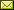## Functorial Manifold Learning

 Dan Shiebler (University of Oxford)

 We adapt previous research on category theory and topological unsupervised learning to develop a functorial perspective on manifold learning, also known as nonlinear dimensionality reduction. We first characterize manifold learning algorithms as functors that map pseudometric spaces to optimization objectives and that factor through hierarchical clustering functors. We then use this characterization to prove refinement bounds on manifold learning loss functions and construct a hierarchy of manifold learning algorithms based on their equivariants. We express several popular manifold learning algorithms as functors at different levels of this hierarchy, including Metric Multidimensional Scaling, IsoMap, and UMAP. Next, we use interleaving distance to study the stability of a broad class of manifold learning algorithms. We present bounds on how closely the embeddings these algorithms produce from noisy data approximate the embeddings they would learn from noiseless data. Finally, we use our framework to derive a set of novel manifold learning algorithms, which we experimentally demonstrate are competitive with the state of the art.

In Kohei Kishida: Proceedings of the Fourth International Conference on Applied Category Theory (ACT 2021), Cambridge, United Kingdom, 12-16th July 2021, Electronic Proceedings in Theoretical Computer Science 372, pp. 1–13.
Published: 3rd November 2022.

 ArXived at: http://dx.doi.org/10.4204/EPTCS.372.1 bibtex PDF
References in reconstructed bibtex, XML and HTML format (approximated).
 Comments and questions to:eptcs@eptcs.org For website issues:webmaster@eptcs.org